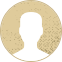Top Special Offer! Check discount

Get 13% off your first order - useTopStart13discount code now!

# Beyond the Numbers: Delving into Calculus

293 views 2 pages ~ 363 words
Get a Custom Essay Writer Just For You!

Experts in this subject field are ready to write an original essay following your instructions to the dot!

For this question, 60% is autonomous (constant) however the remaining 40% (that is, 100 – 60 = 40) depends on the ratio of time spent studying. This can be modeled by a linear equation of the form

Y = 60 + 40X

where; Y- average score and X- the ratio of time spent studying.

Given X = 0.5 (half) Y is obtained as.

Y = 60 + 0.5*40 = 80%.

Assuming I wanted a score of 95% then

95 = 60 +40X

X = 7/8 implying that I should study for at least 7/8 of the study time.

Question 2

In this question 30% is guaranteed, however, the remaining 50% (that is 80 – 30 =50) depends on the ratio of time spent studying. This can be modeled by a linear equation of the form

Y = 30 + 50X

where; Y- average score and X- the ratio of time spent studying.

Given X = 0.5 (half) Y is obtained as.

Y = 30 + 0.5*50 = 55%.

Assuming I wanted a score of 70% then

70 = 30 +50X

X = 4/5 implying that I should study for at least 4/5 of the time.

Question 3

Given probability of passing p = 0.6

Expected yards’ offense will gain from passing (E1) is obtained as follows:

E1

= 4*(0.6) + 9*(1-0.6)

= 2.4 + 3.6

= 6 yards

Expected yards’ offense will gain from run (E2) is obtained as follows:

E1

= 6*(0.6) + 1*(1-0.6)

= 3.6 + 0.4

= 4 yards

Therefore, the average yards the offense  can expect to gain is  = 5 yards.

Question 4

Given probability of passing p = 0.75

Expected yards’ defense will gain from passing (E3) is obtained as follows:

E3

= 2*(0.75) + 15*(1-0.75)

= 1.5 + 3.75

= 5.25 yards

Expected yards’ defense will gain from run (E4) is obtained as follows:

E4

= 5*(0.75) + 0*(1-0.75)

= 3.75 yards

Since the defense gain less yards for every run my next play will be a run

Question 5

#1 Confesses

# 1 Stays Silent

#2 Confesses

-5 years

-10 years

#2 Stays Silent

0 years

-6 months

The decision, in this case, will depend on the worst case scenario for the suspect. If #1 confess the worst can be 6 months in prison and the best can be 0 months. While if one stays silent the worst case can be 10 years and best 6 months. Therefore, suspect number one should confess.

Question 6

The odds of winning is 0.04 or 4 % (Multi-State Lottery Association). Therefore, expected payoff of a single lottery is 0.04*2 = \$0.08. When the expected payoff is at least half of the ticket price. That is 50% chance of winning.

Work Cited

Multi-State Lottery Association. Powerball. October 2015. HTML. 12 February 2018. <http://www.powerball.com/powerball/pb_howtoplay.asp>.

September 18, 2023
Category:
Subcategory:
Subject area:
Number of pages

2

Number of words

363

59Rate:

5

Expertise Calculus
Verified writer

LuckyStrike has helped me with my English and grammar as I asked him for editing and proofreading tasks. When I need professional fixing of my papers, I contact my writer. A great writer who will make your writing perfect.

Hire Writer

This sample could have been used by your fellow student... Get your own unique essay on any topic and submit it by the deadline.

Eliminate the stress of Research and Writing!

Hire one of our experts to create a completely original paper even in 3 hours!